# 2. (20 points) Using the unit load method (virtual work), find the horizontal displacement of node...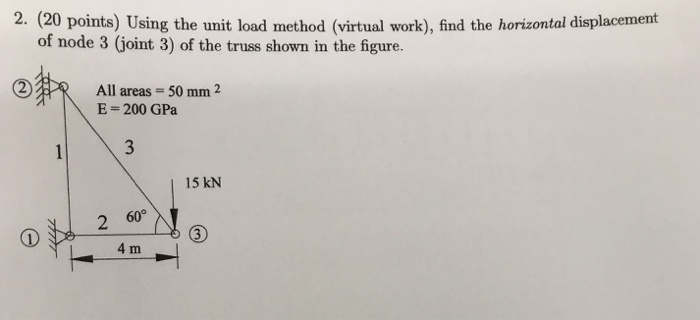2. (20 points) Using the unit load method (virtual work), find the horizontal displacement of node 3 (joint 3) of the truss shown in the figure. ? R All areas = 50 mm 2 E = 200 GPa 15 kN 2 60 L4 m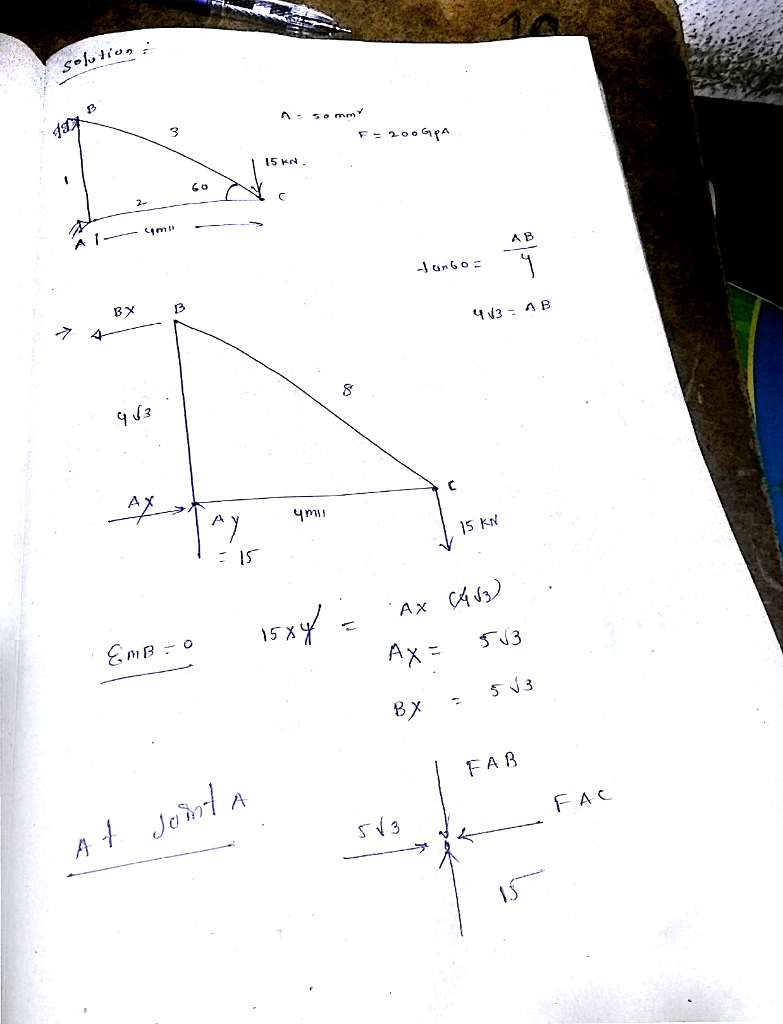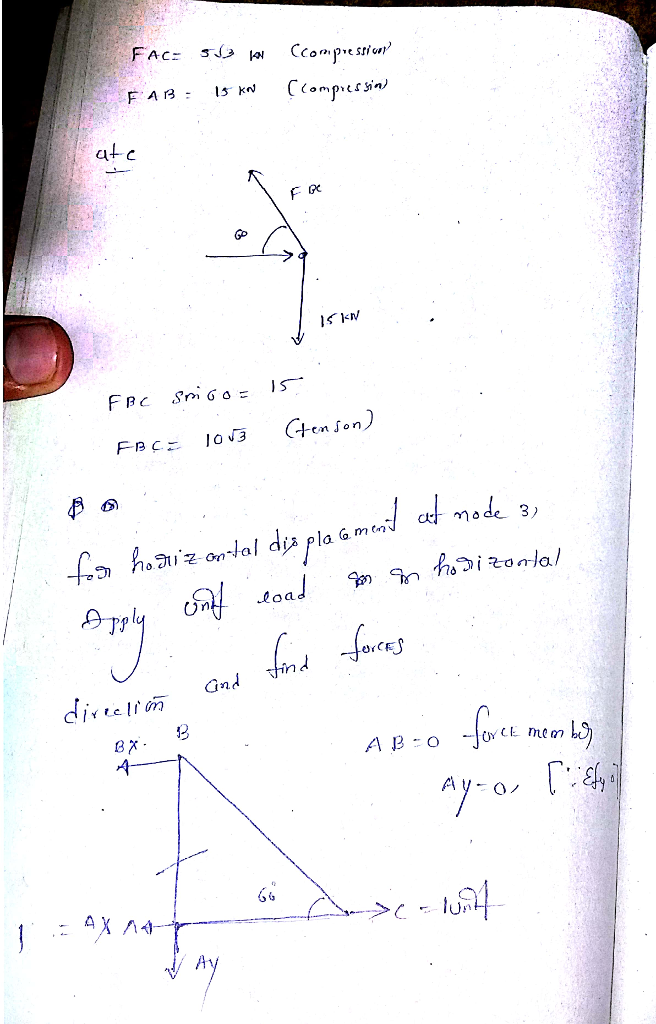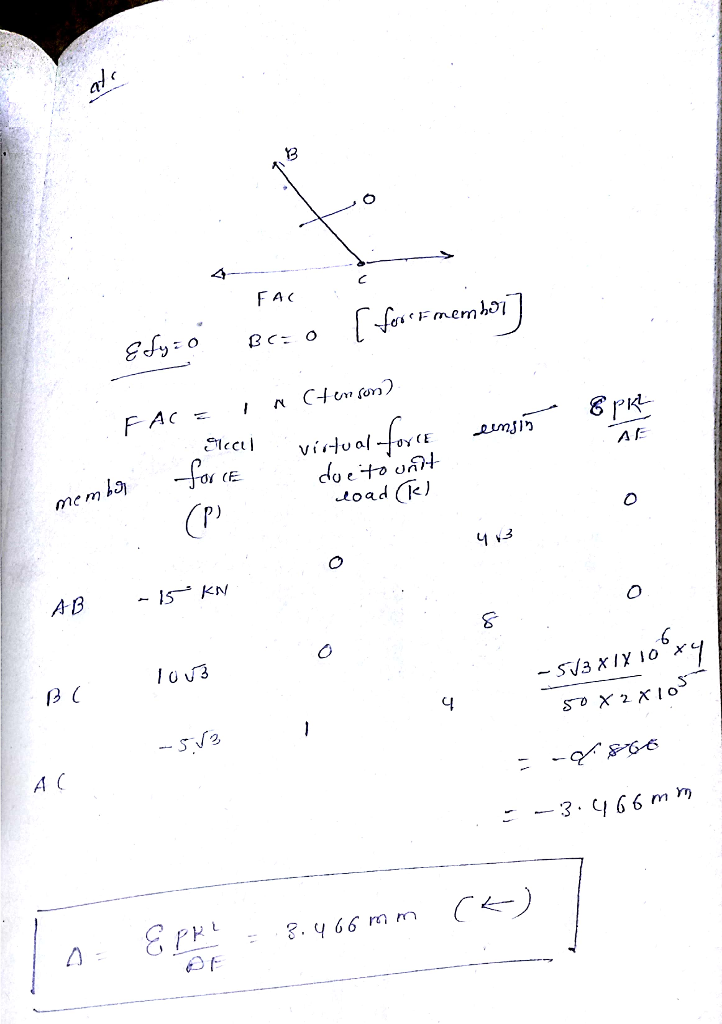#### Earn Coin

Coins can be redeemed for fabulous gifts.

Similar Homework Help Questions
• ### Q3: Determine the vertical and horizontal displacement of joint A for the truss shown in Fig....Q3: Determine the vertical and horizontal displacement of joint A for the truss shown in Fig. (3). each bar is made of steel and has the cross-sectional area of 400mm Take E = 200 GPa Use the method of virtual work. E D 2 m |в -1.5 m -1.5 m 20 KN 40 KN Fig. (3)

• ### 2. Determine the vertical displacement at joint B and horizontal displacement at joint D using Castigliano's...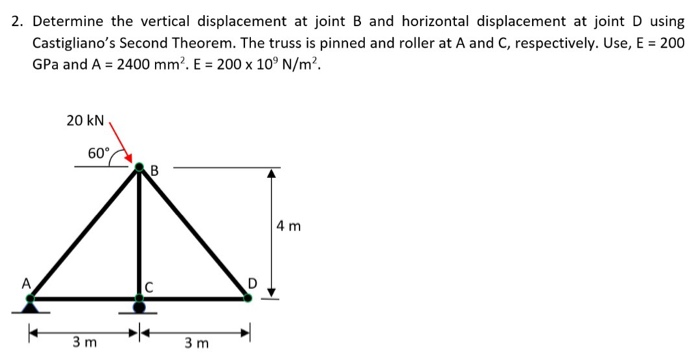2. Determine the vertical displacement at joint B and horizontal displacement at joint D using Castigliano's Second Theorem. The truss is pinned and roller at A and C, respectively. Use, E = 200 GPa and A = 2400 mm. E = 200 x 10°N/m². 20 KN 60 CAB 4 m 3m 3m

• ### Using the virtual work method, determine the horizontal deflection at joint C of the truss. of...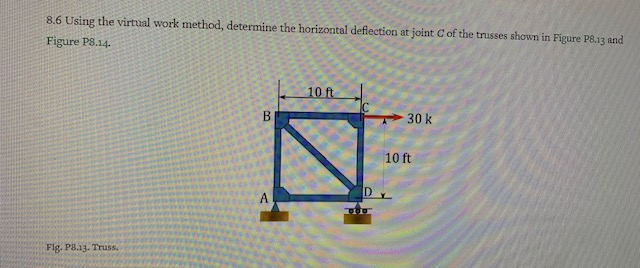Using the virtual work method, determine the horizontal deflection at joint C of the truss. of the trusses shown in Figure P8.13 and 8.6 Using the virtual work method, determine the horizontal deflection at joint Figure P8.14 101 30 k 10 ft Fig. 3.13. Truss.

• ### Q3: Determine the vertical and horizontal displacement of joint A for the truss shown in Fig....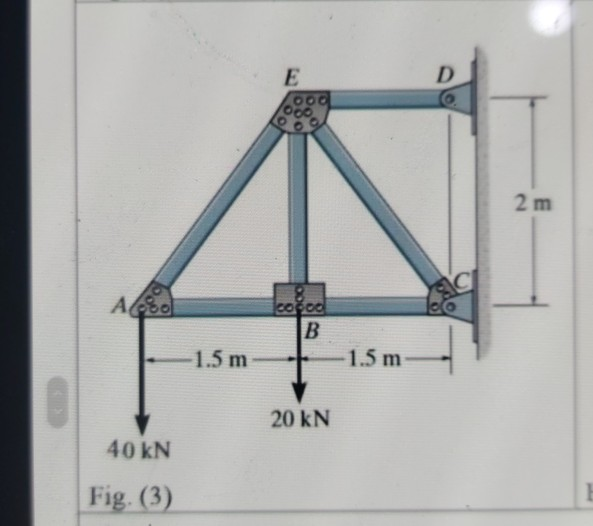Q3: Determine the vertical and horizontal displacement of joint A for the truss shown in Fig. (3), each bar is made of steel and has the cross-sectional area of 400mm?. Take E = 200 GPa. Use the method of virtual work. E D 00. 2 m СІ Ao cocoa B 1.5 m 1.5 m 20 KN 40 kN Fig. (3)

• ### The plane truss is subjected to a load as shown in Figure 4. Take E = 200 GPa and cross sectional areas of members 1, 2...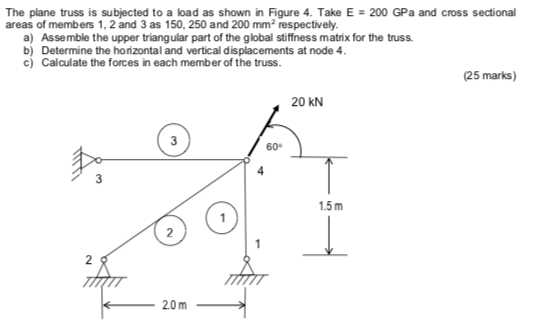The plane truss is subjected to a load as shown in Figure 4. Take E = 200 GPa and cross sectional areas of members 1, 2 and 3 as 150, 250 and 200 mm2 respectively a) Assemble the upper triangular part of the global stiffness matrix for the truss b) Determine the horizontal and vertical displacements at node 4 c) Calculate the forces in each member of the truss. (25 marks) 20 kN 3 60° 4 1.5m 2 2 20m...

• ### i need the answer quickly fast as you can Q3: Determine the vertical and horizontal displacement...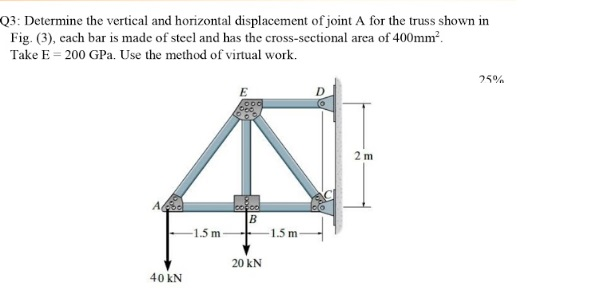i need the answer quickly fast as you can Q3: Determine the vertical and horizontal displacement of joint A for the truss shown in Fig. (3), cach bar is made of steel and has the cross-sectional area of 400mm? Take E = 200 GPa. Use the method of virtual work. 25% E 2 m А. cool B 1.5 m -1.5 m 20 KN 40 KN

• ### Portalio Problems-Virtal Work M ork Method Problem 3 For the frame structure below: a) Use the me...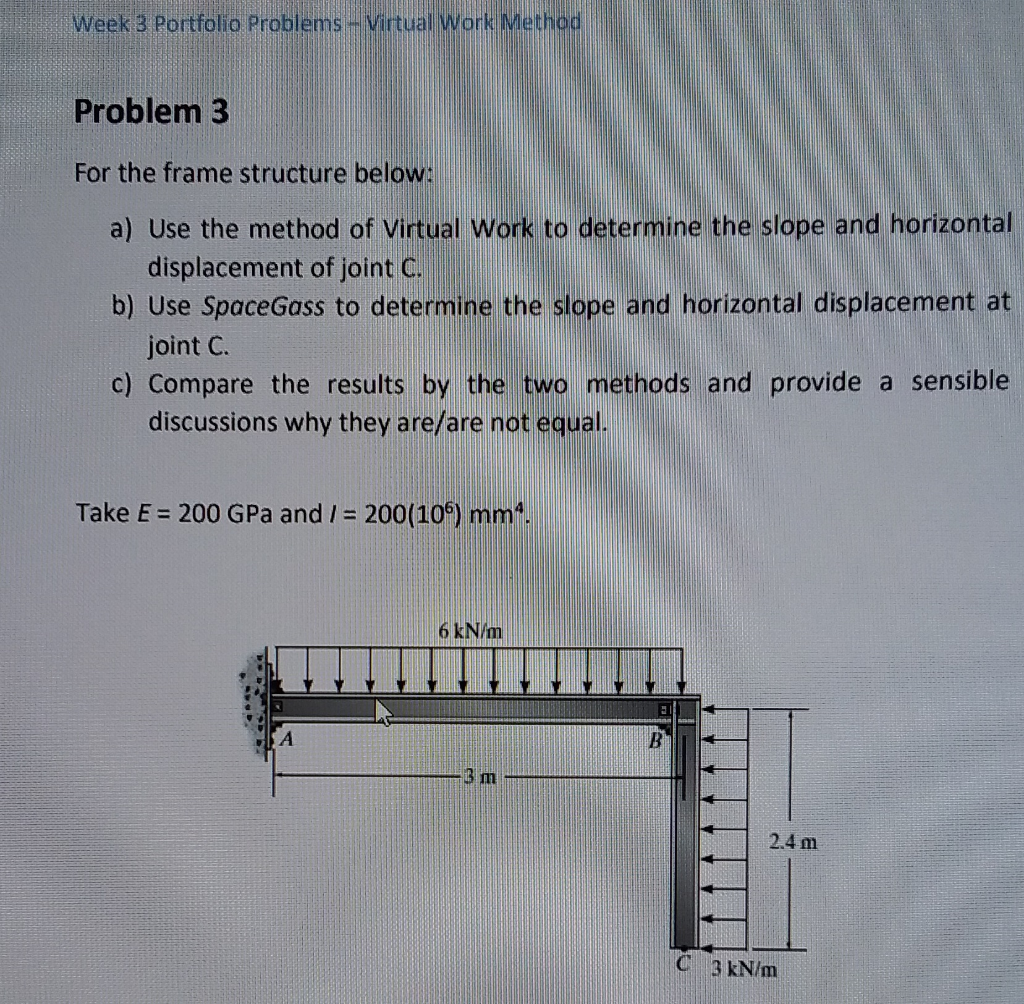Portalio Problems-Virtal Work M ork Method Problem 3 For the frame structure below: a) Use the method of Virtual Work to determine the slope and horizontal b) Use SpaceGass to determine the slope and horizontal displacement at c) Compare the results by the two methods and provide a sensible displacement of joint C joint C discussions why they are/are not equal. Take E 200 GPa and /- 200(10) mm 6 kN/m 3 m 2.4 m C 3 kN/m Portalio Problems-Virtal...

• ### For truss shown below a vertical load of 25 KN and Horizontal Load of 30 KN...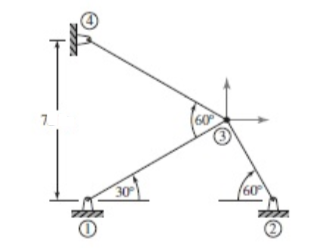For truss shown below a vertical load of 25 KN and Horizontal Load of 30 KN applied at Node 3 ( Use FEM Nodal displacement, Direct stiffness method) 1). Calculate clearly the member length and distance between members A = 5 x 10^-4 m^2 and E = 200 GPa 2). Determine the member and global stiffness matrix and show the calculation fot Sinθ and Cosθ clearly 3). determine the displacement and member forces All Load and dimensions are in meter...

• ### Determine the horizontal displacement at the node where 20 kN is applied. Also compute the reaction forces at the suppor...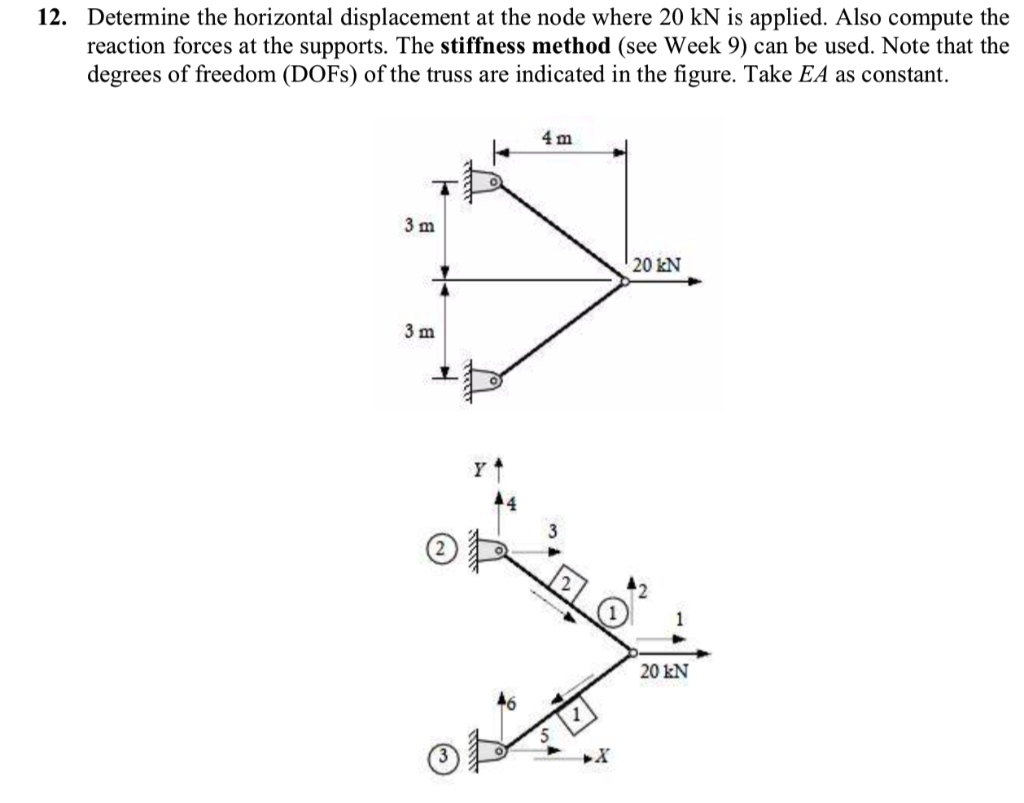Determine the horizontal displacement at the node where 20 kN is applied. Also compute the reaction forces at the supports. The stiffness method (see Week 9) can be used. Note that the degrees of freedom (DOFs) of the truss are indicated in the figure. Take EA as constant. 12. Determine the horizontal displacement at the node where 20 kN is applied. Also compute the reaction forces at the supports. The stiffness method (see Week 9) can be used. Note that...

• ### Determine the horizontal displacement at A. Take E = 200 GPa. The moment of inertia of...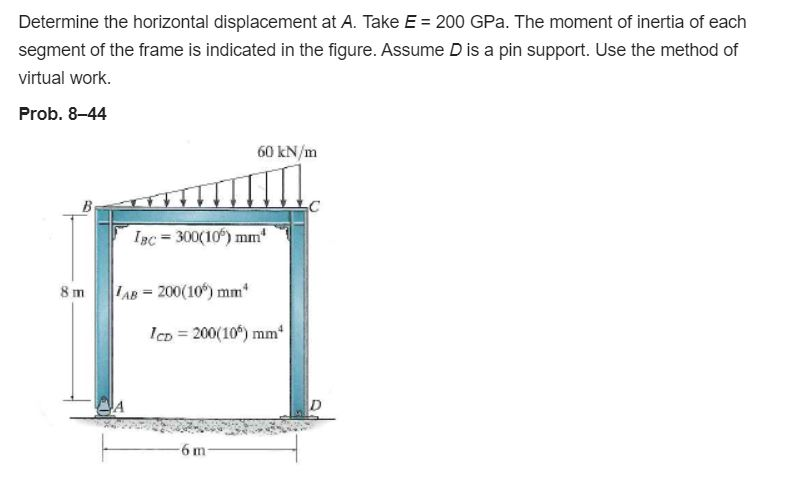Determine the horizontal displacement at A. Take E = 200 GPa. The moment of inertia of each segment of the frame is indicated in the figure. Assume D is a pin support. Use the method of virtual work. Prob. 8-44 60 kN/m B 1pc = 300(10%) mm 8 m AB= 200(106) mm IcD = 200(10%) mm 6 m

Free Homework Help App